Function Repository Resource:

# EvolutoidCurve

Compute the evolutoid of a curve

Contributed by: Jan Mangaldan
 ResourceFunction["EvolutoidCurve"][c,θ,t] computes the evolutoid with angle θ of the curve c defined with respect to the variable t.

## Details

An evolutoid is the envelope of all the lines that intersect a plane curve and are at a constant angle θ from the curve's tangents.
When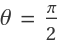, the evolute is obtained.

## Examples

### Basic Examples (3)

Parametric equations for an ellipse:

 In:=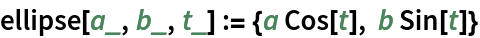Compute the evolutoid of an ellipse with angle equal to GoldenAngle:

 In:=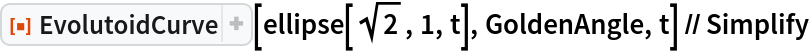Out=Plot the ellipse and its evolutoid:

 In:=Out=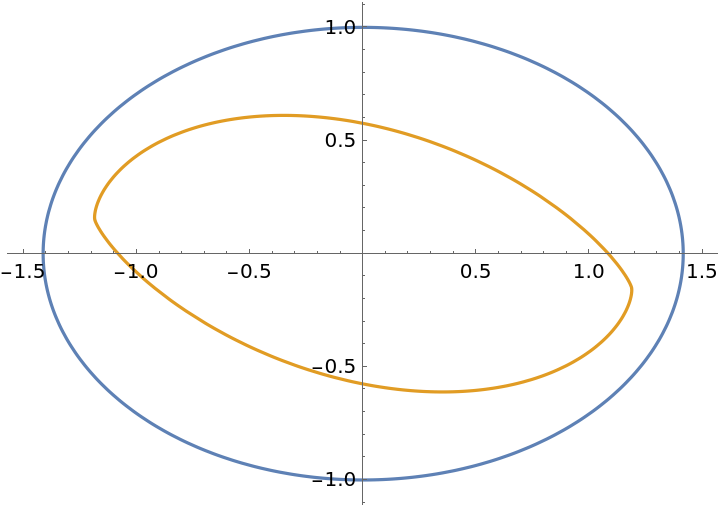### Properties and Relations (2)

When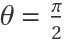, EvolutoidCurve is the same as the resource function EvoluteCurve:

 In:=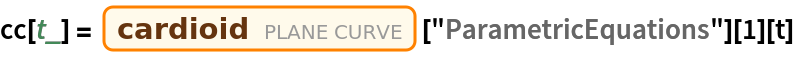Out=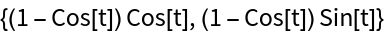In:=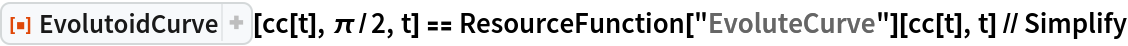Out=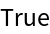Demonstrate the effect of changing θ in EvolutoidCurve:

 In:=Out=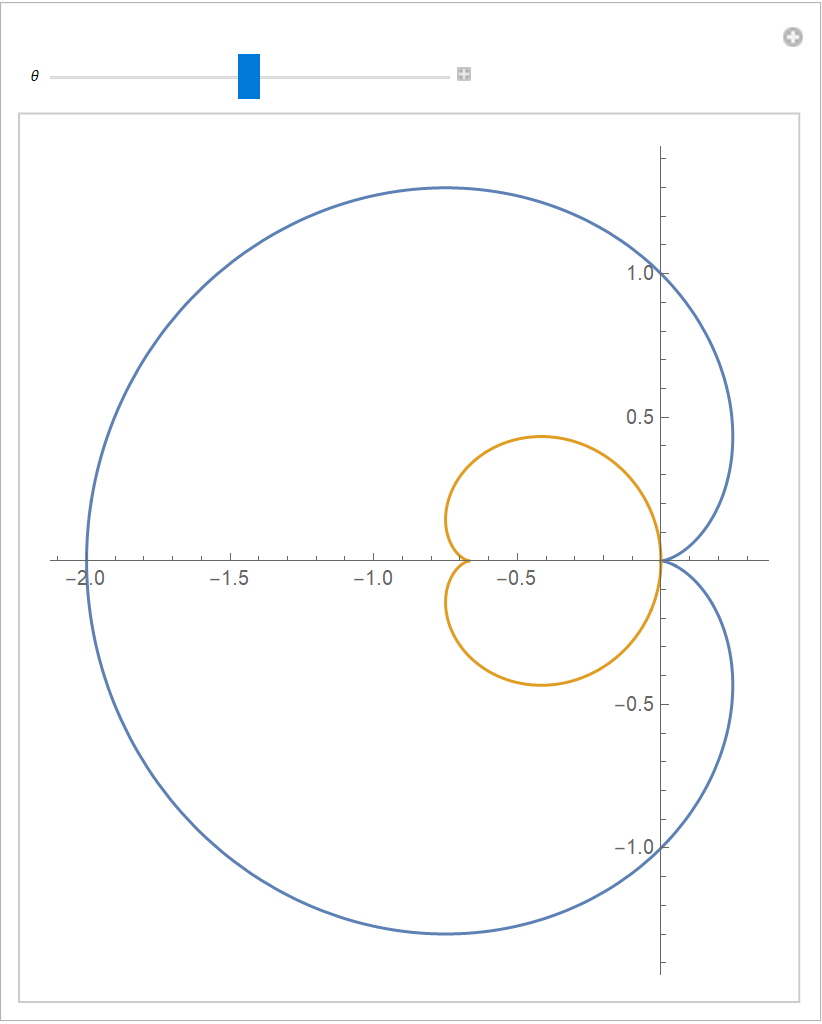## Version History

• 1.0.0 – 11 February 2021